# ML 109: Poisoning Labels with SecML (30 pts extra)

## What You Need

• A Web browser

## Purpose

To practice using SecML, an open-source Python library for the security evaluation of Machine Learning algorithms.

In a browser, go to

From the menu, click File, "New notebook".

## Installing SecML

Execute these commands:
```!pip install secml import secml ```
The library installs, as shown below.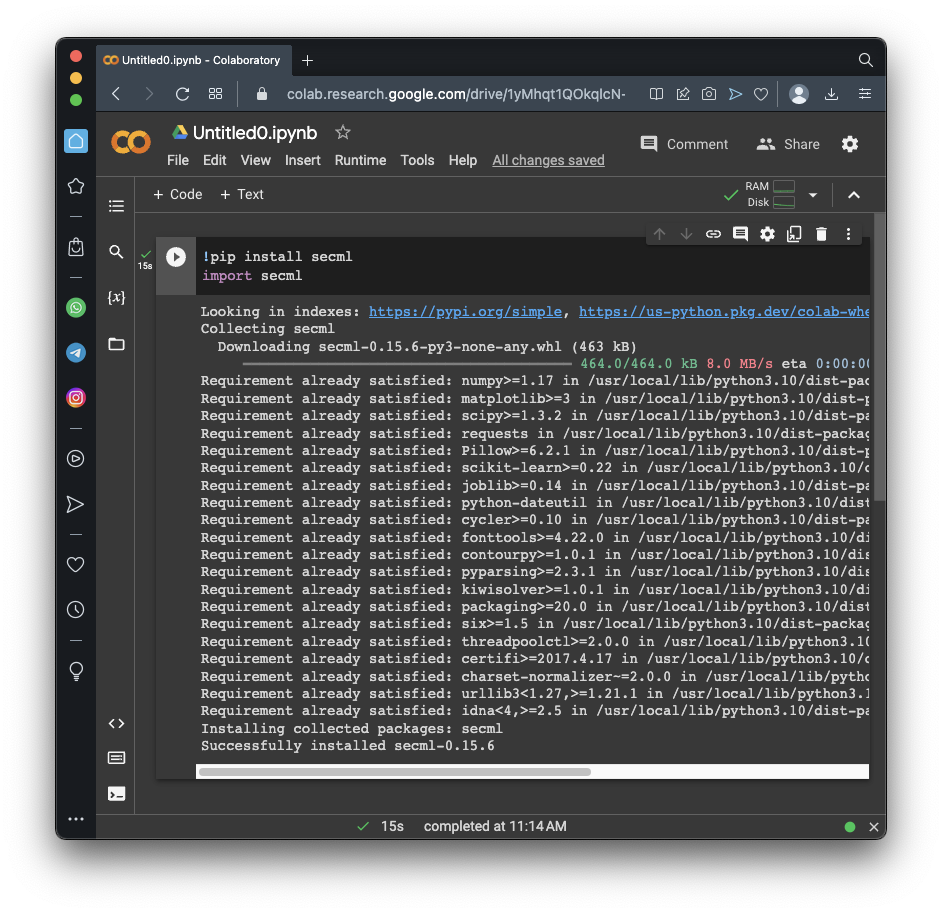## Preparing a Dataset

Execute these commands to create a simple, artificial dataset consisting of three groups of points in a plane.

We need training and testing sets, as usual. The validation set isn't used in this project, but it will be in the next one.

```random_state = 999 n_features = 2 # Number of features n_samples = 300 # Number of samples centers = [[-1, -1], [1, 1]] # Centers of the clusters cluster_std = 0.9 # Standard deviation of the clusters from secml.data.loader import CDLRandomBlobs dataset = CDLRandomBlobs(n_features=n_features, centers=centers, cluster_std=cluster_std, n_samples=n_samples, random_state=random_state).load() n_tr = 100 # Number of training set samples n_val = 100 # Number of validation set samples n_ts = 100 # Number of test set samples # Split in training, validation and test from secml.data.splitter import CTrainTestSplit splitter = CTrainTestSplit( train_size=n_tr + n_val, test_size=n_ts, random_state=random_state) tr_val, ts = splitter.split(dataset) splitter = CTrainTestSplit( train_size=n_tr, test_size=n_val, random_state=random_state) tr, val = splitter.split(dataset) # Normalize the data from secml.ml.features import CNormalizerMinMax nmz = CNormalizerMinMax() tr.X = nmz.fit_transform(tr.X) val.X = nmz.transform(val.X) ts.X = nmz.transform(ts.X) # Display the training set from secml.figure import CFigure # Only required for visualization in notebooks %matplotlib inline fig = CFigure(width=5, height=5) # Convenience function for plotting a dataset fig.sp.plot_ds(tr) fig.show() ```
As shown below, you see two categories of dots, shown in different colors.

The task of this model is to sort the dots into their categories.## Creating and Training the Model

Execute these commands to create and train the model:
```# Metric to use for training and performance evaluation from secml.ml.peval.metrics import CMetricAccuracy metric = CMetricAccuracy() # Creation of the multiclass classifier from secml.ml.classifiers import CClassifierSVM from secml.ml.kernels import CKernelRBF clf = CClassifierSVM(kernel=CKernelRBF(gamma=10), C=1) # We can now fit the classifier clf.fit(tr.X, tr.Y) print("Training of classifier complete!") # Compute predictions on a test set y_pred = clf.predict(ts.X) # Evaluate the accuracy of the classifier acc = metric.performance_score(y_true=ts.Y, y_pred=y_pred) print("Accuracy on test set: {:.2%}".format(acc)) ```
As shown below, the model has a 94% accuracy.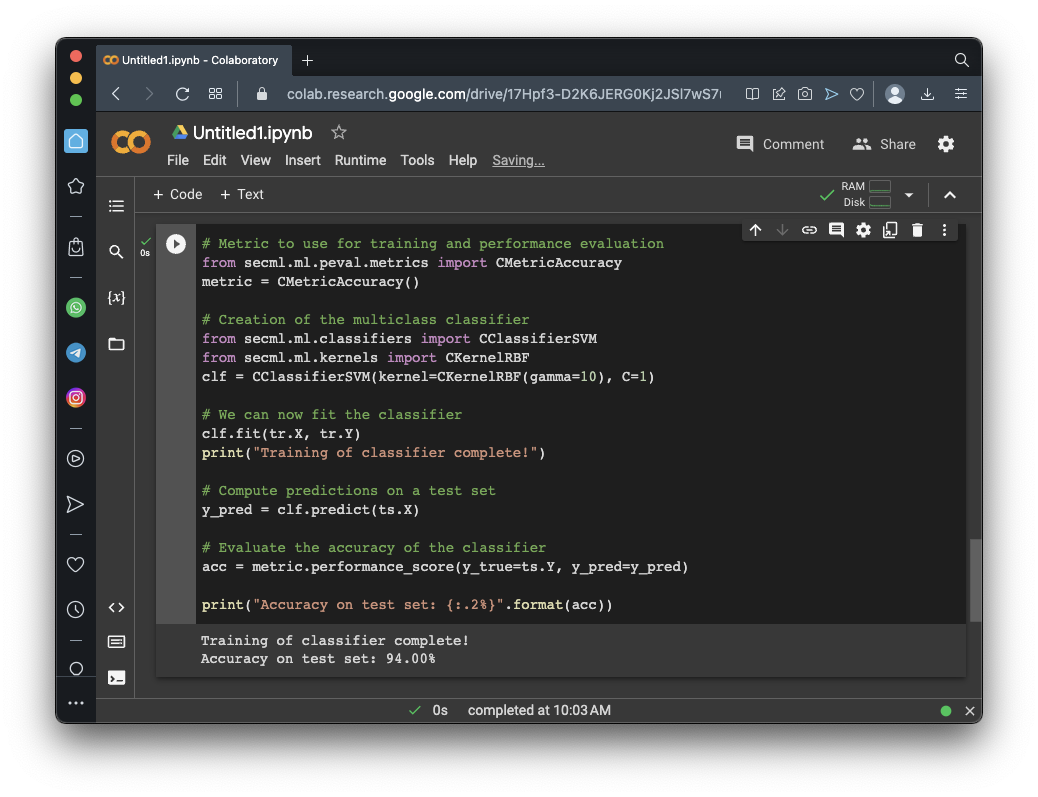## Visualizing the Results

Execute these commands to see a pretty cart showing the regions the model classifies data into:
```fig = CFigure(width=5, height=5) # Convenience function for plotting the decision function of a classifier fig.sp.plot_decision_regions(clf, n_grid_points=200) fig.sp.plot_ds(ts) fig.sp.grid(grid_on=False) fig.sp.title("Classification regions") fig.sp.text(0.01, 0.01, "Accuracy on test set: {:.2%}".format(acc), bbox=dict(facecolor='white')) fig.show() ```
As shown below, the model draws a curve between the regions, more or less the same way a human would.## Manipulating One Label

This is the simplest poisoning attack: changing the data labels to confuse the model.

Execute these commands to change the first label:

```print("Training set:", tr.Y) tr_poisoned = tr.deepcopy() tr_poisoned.Y = 0 print("Poisoned: ", tr_poisoned.Y) print('X: ({:.2f}, {:.2f})'.format(tr_poisoned.X.tolist(), tr_poisoned.X.tolist())) print() fig = CFigure(width=9, height=4) fig.subplot(1, 2, 1) fig.sp.plot_ds(tr) fig.subplot(1, 2, 2) fig.sp.plot_ds(tr_poisoned) fig.show() ```
The chart on the left shows the original data, and the chart on the right shows the poisoned data.

As shown below, one red dot in the top center is now blue.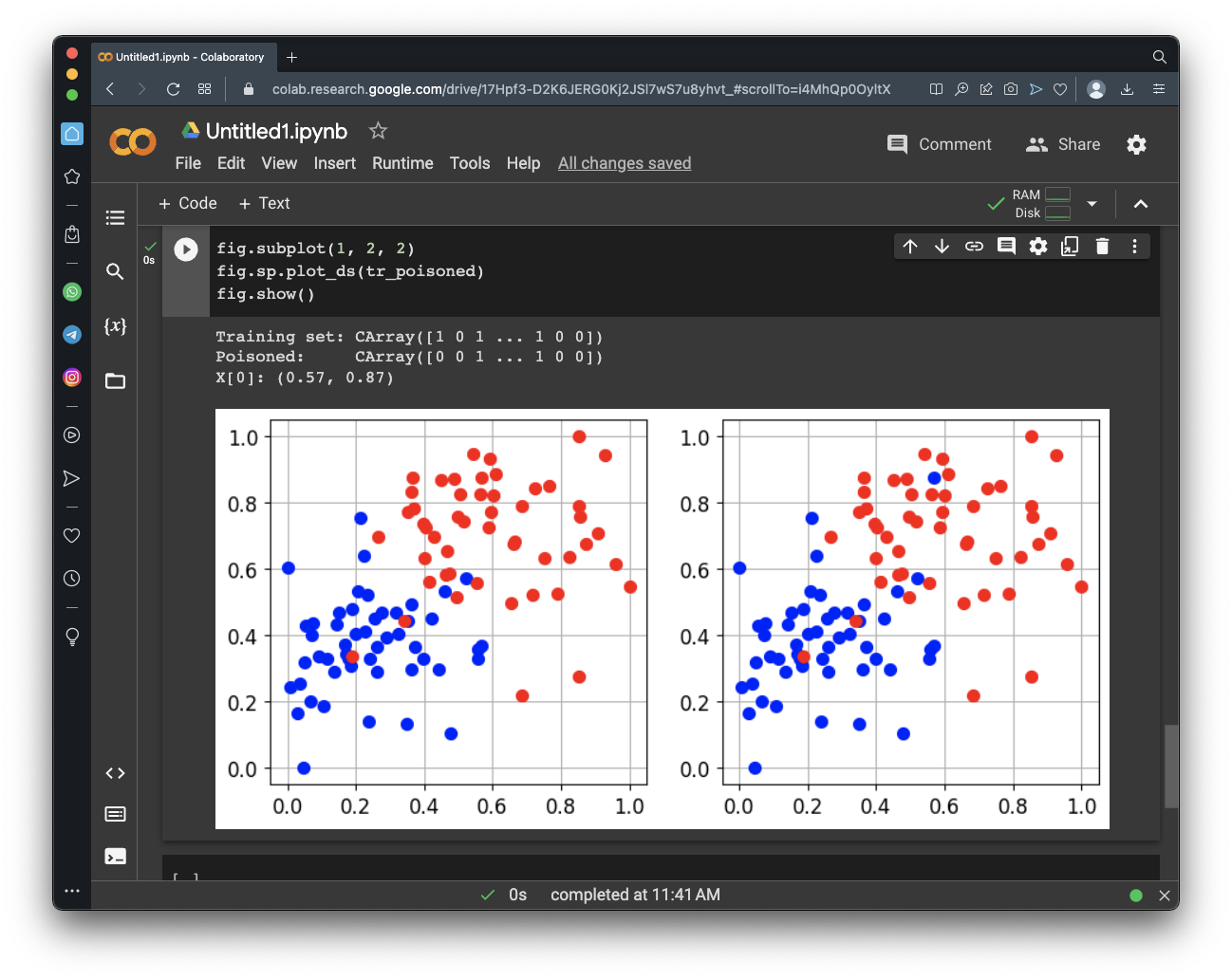## Performance of Poisoned Model

Execute these commands to create and train the poisoned model:
``` # Create the poisoned multiclass classifier clf_poisoned = CClassifierSVM(kernel=CKernelRBF(gamma=10), C=1) # Fit the poisoned classifier clf_poisoned.fit(tr_poisoned.X, tr_poisoned.Y) print("Training of poisoned classifier complete!") # Compute predictions on a test set y_pred_poisoned = clf_poisoned.predict(ts.X) # Evaluate the accuracy of the classifier acc_poisoned = metric.performance_score(y_true=ts.Y, y_pred=y_pred_poisoned) print("Accuracy on test set before poisoning: {:.2%}".format(acc)) print("Accuracy on test set after poisoning: {:.2%}".format(acc_poisoned)) ```
As shown below, the model has a 94% accuracy.## Manipulating More Labels

Execute these commands to loop through the first 40 dots, and change all the red dots to blue:
```print("Training set:", tr.Y) tr_poisoned = tr.deepcopy() for i in range(40): if tr_poisoned.Y[i] == 1: tr_poisoned.Y[i] = 0 # else: # tr_poisoned.Y[i] = 1 print("Poisoned: ", tr_poisoned.Y) print('X: ({:.2f}, {:.2f})'.format(tr_poisoned.X.tolist(), tr_poisoned.X.tolist())) print() fig = CFigure(width=9, height=4) fig.subplot(1, 2, 1) fig.sp.plot_ds(tr) fig.subplot(1, 2, 2) fig.sp.plot_ds(tr_poisoned) fig.show() ```
The chart on the left shows the original data, and the chart on the right shows the poisoned data.

As shown below, many red dots have turned blue.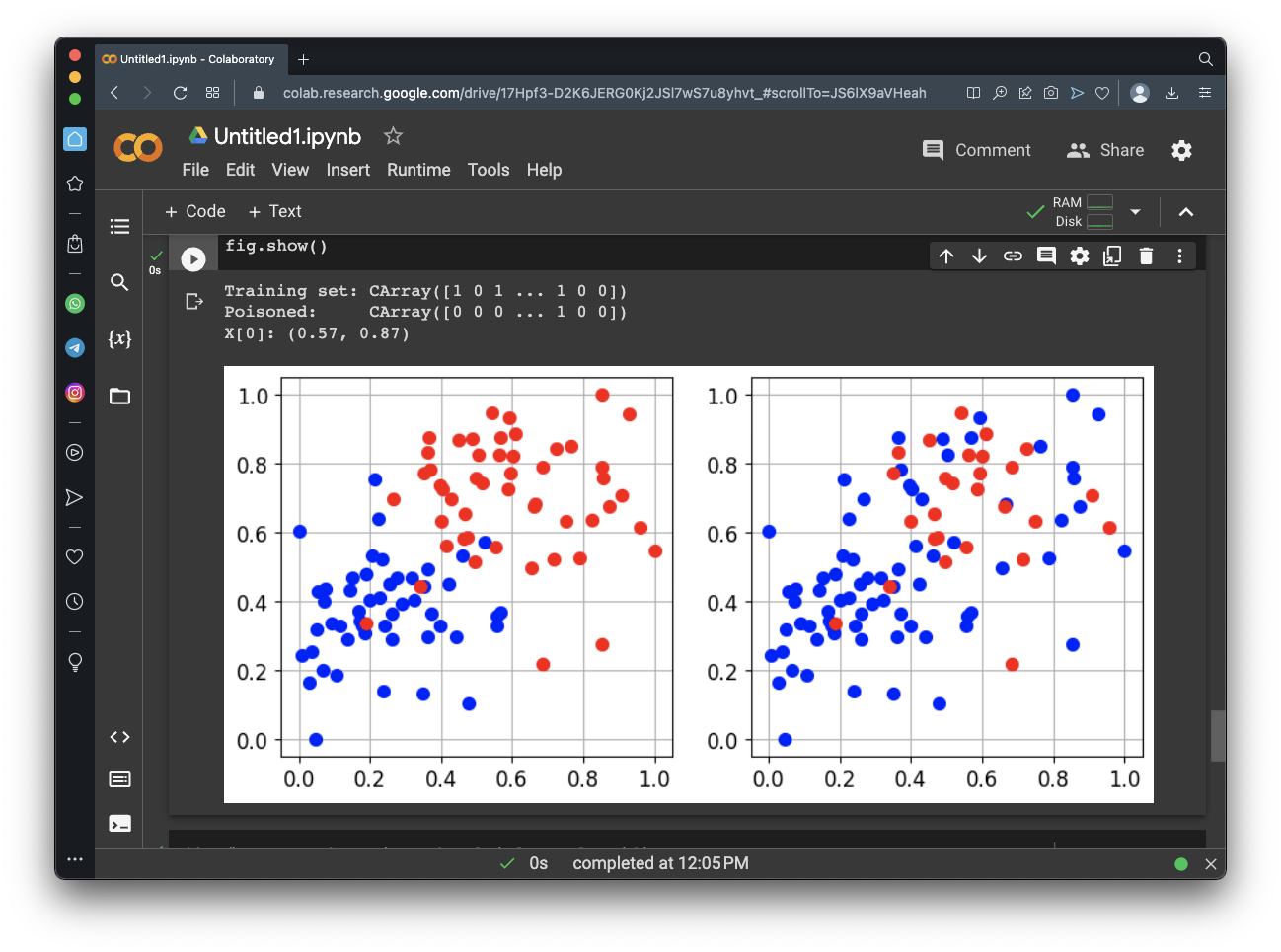## Performance of Poisoned Model

Execute these commands to create and train the poisoned model:
```# Create the poisoned multiclass classifier clf_poisoned = CClassifierSVM(kernel=CKernelRBF(gamma=10), C=1) # Fit the poisoned classifier clf_poisoned.fit(tr_poisoned.X, tr_poisoned.Y) print("Training of poisoned classifier complete!") # Compute predictions on a test set y_pred_poisoned = clf_poisoned.predict(ts.X) # Evaluate the accuracy of the classifier acc_poisoned = metric.performance_score(y_true=ts.Y, y_pred=y_pred_poisoned) print("Accuracy on test set before poisoning: {:.2%}".format(acc)) print("Accuracy on test set after poisoning: {:.2%}".format(acc_poisoned)) ```
As shown below, poisoning has lowered the accuracy to 74%.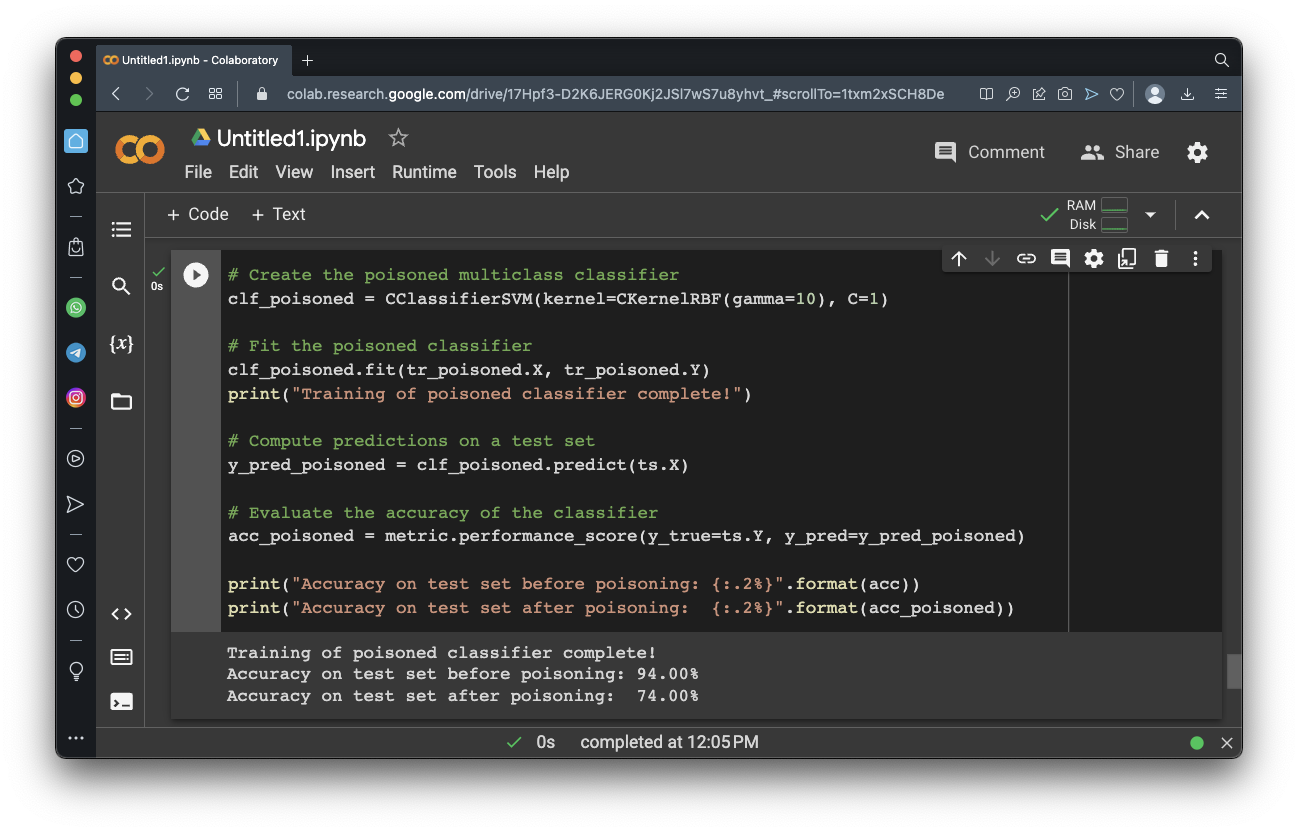## Flag ML 109.1: Poisoning Even More Red Dots (10 pts)

Adjust the attack above to test the first 60 dots in the training set, and turn the red dots blue.

The flag is covered by a green rectangle in the image below.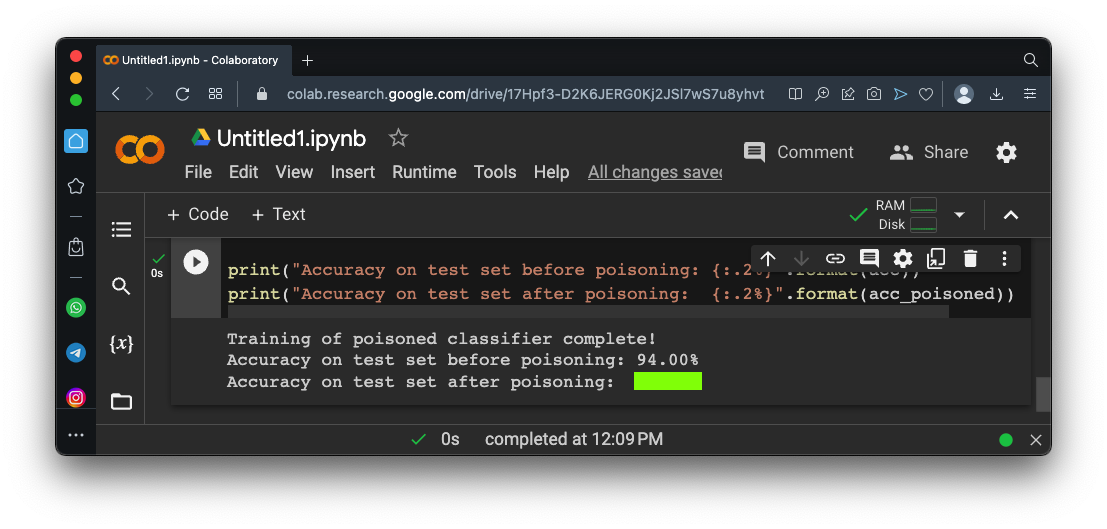## Flag ML 109.2: Reversing Dots (10 pts)

Adjust the attack above to reverse the colors of the first 40 dots in the training set, as shown below.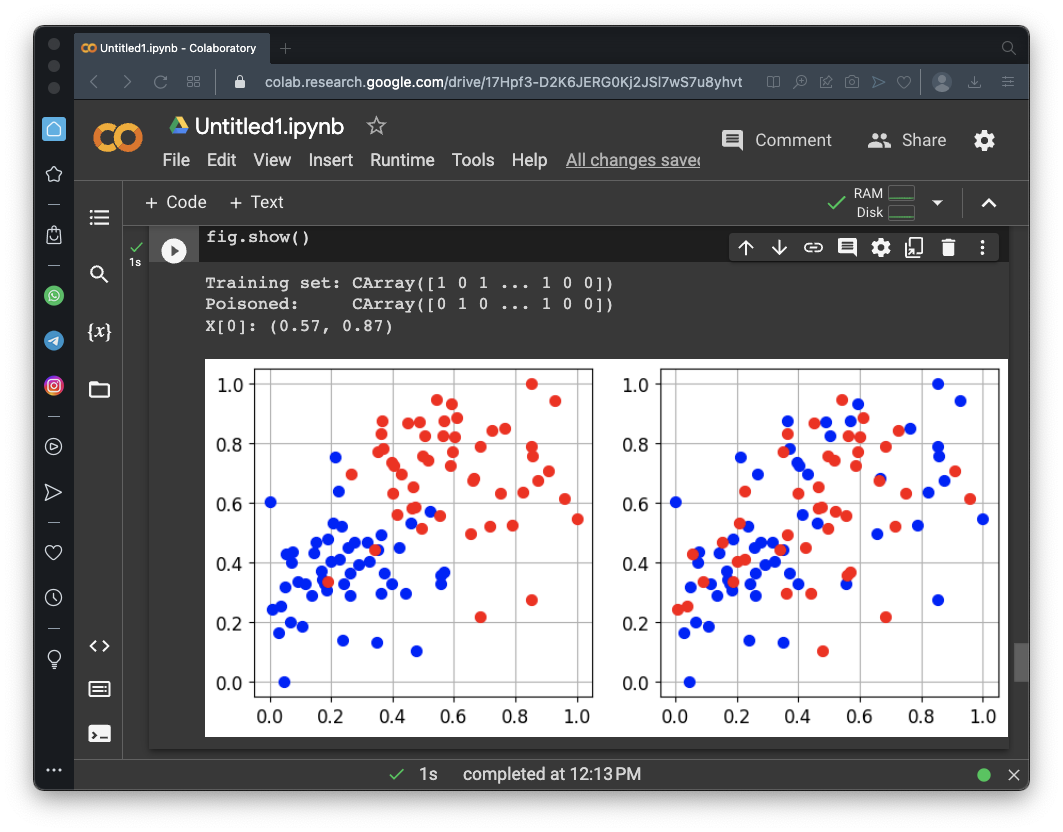The flag is covered by a green rectangle in the image below.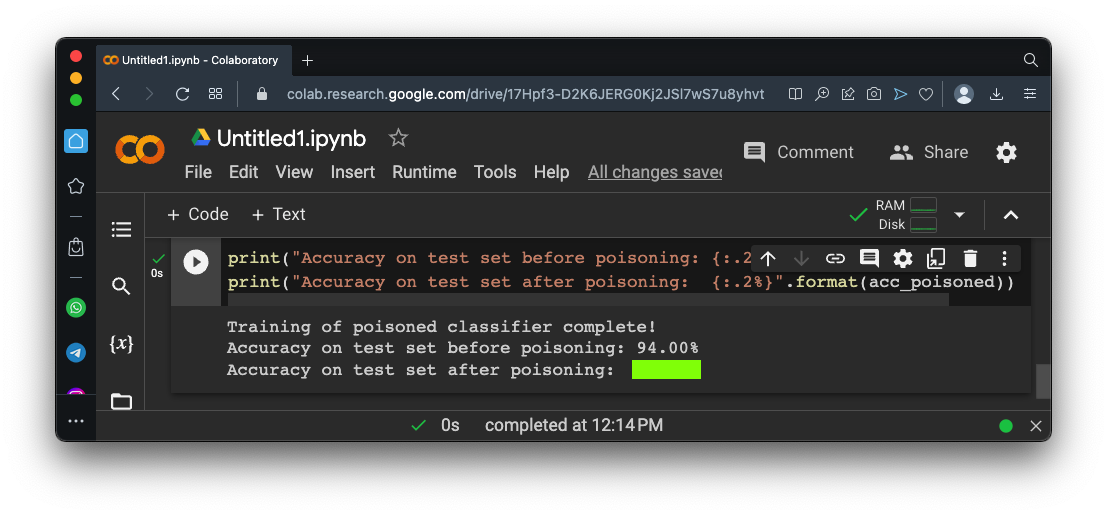## Flag ML 109.3: Poisoning Rightmost Red Dots (10 pts)

Adjust the attack above to change the red dots with a horizontal coordinate greater than 0.8 blue, as shown below.The flag is covered by a green rectangle in the image below.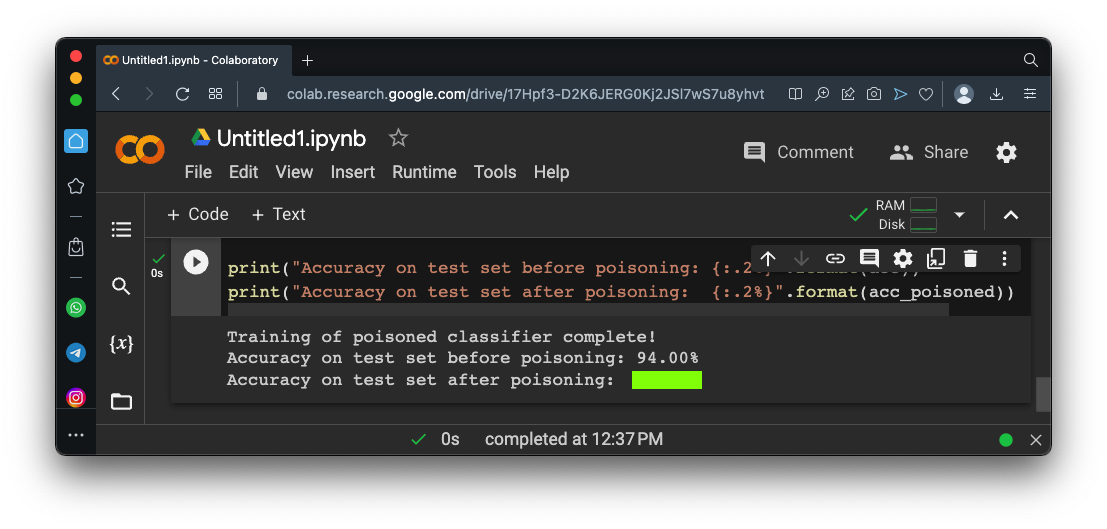## Sources

SecML: Secure and Explainable Machine Learning in Python
Poisoning Attacks against Machine Learning models
Poisoning attacks on Machine Learning

Posted 5-4-23